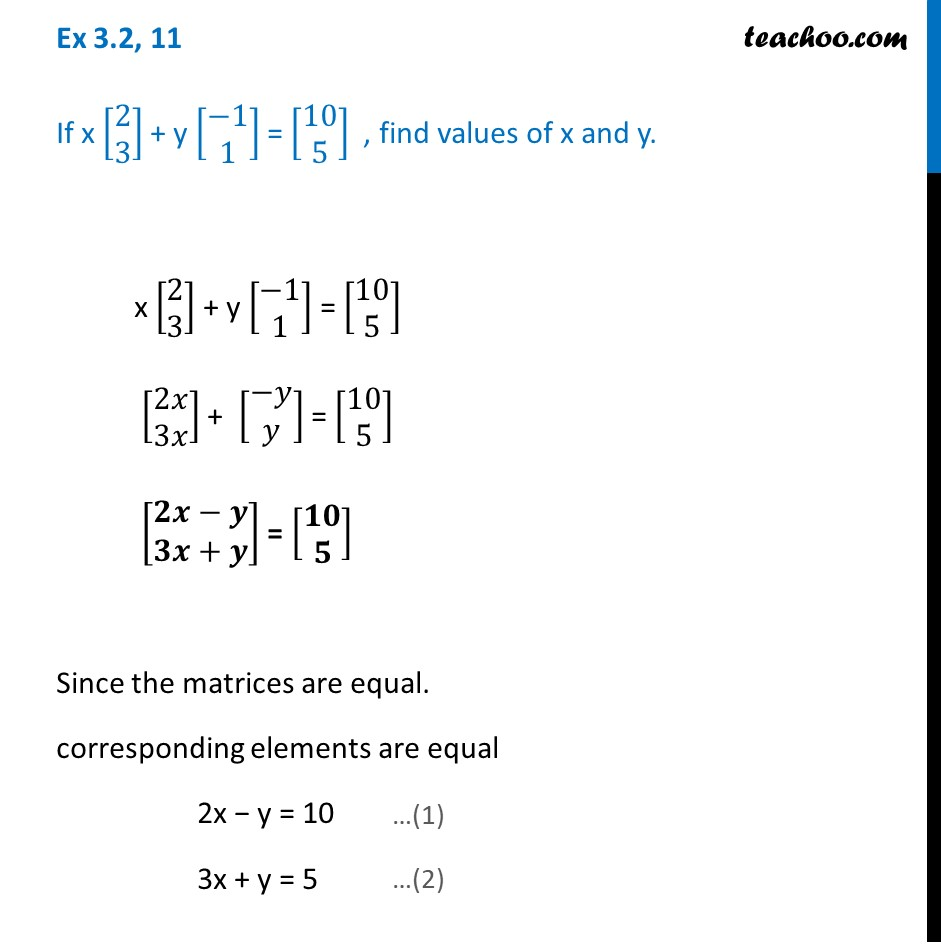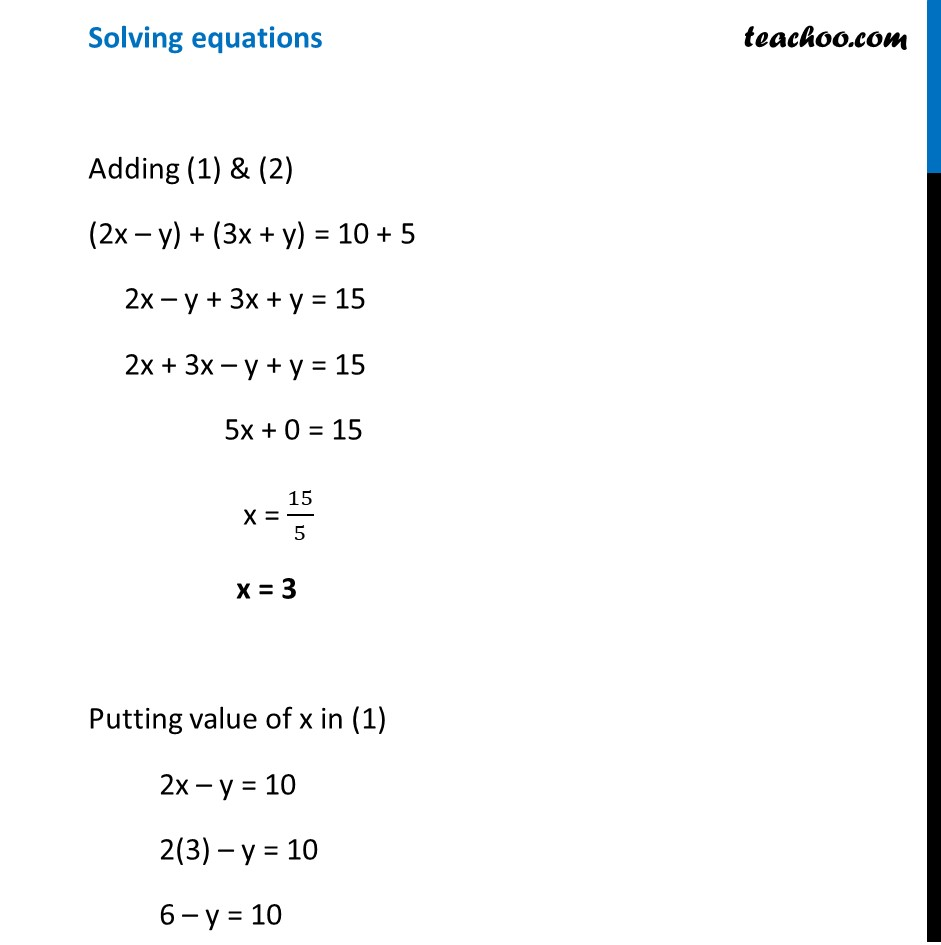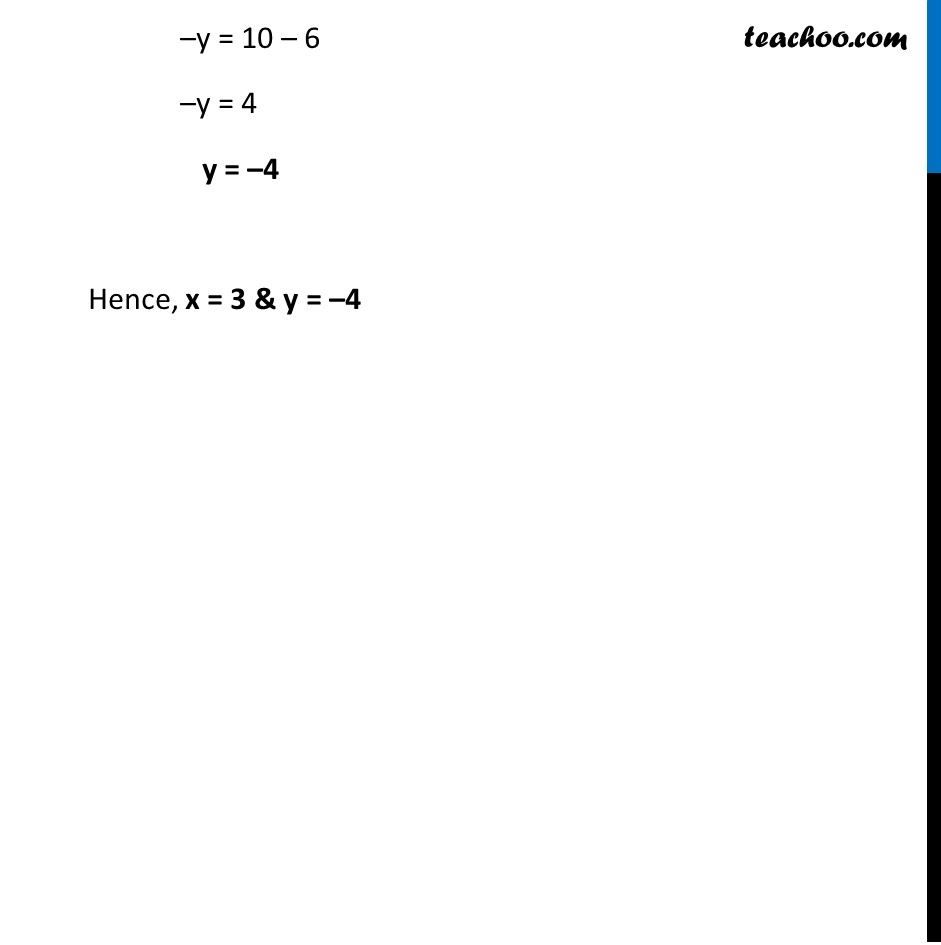Chapter 3 Class 12 Matrices
Concept wiseLearn in your speed, with individual attention - Teachoo Maths 1-on-1 Class

### Transcript

Ex 3.2, 11 If x [■8(2@3)] + y [■8(−1@1)] = [■8(10@5)] , find values of x and y. x [■8(2@3)] + y [■8(−1@1)] = [■8(10@5)] [■8(2𝑥@3𝑥)] + [■8(−𝑦@𝑦)] = [■8(10@5)] [■8(𝟐𝒙−𝒚@𝟑𝒙+𝒚)] = [■8(𝟏𝟎@𝟓)] Since the matrices are equal. corresponding elements are equal 2x − y = 10 3x + y = 5 Solving equations Adding (1) & (2) (2x – y) + (3x + y) = 10 + 5 2x – y + 3x + y = 15 2x + 3x – y + y = 15 5x + 0 = 15 x = 15/5 x = 3 Putting value of x in (1) 2x – y = 10 2(3) – y = 10 6 – y = 10 –y = 10 – 6 –y = 4 y = –4 Hence, x = 3 & y = –4